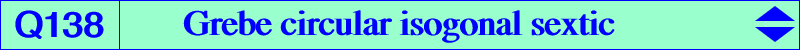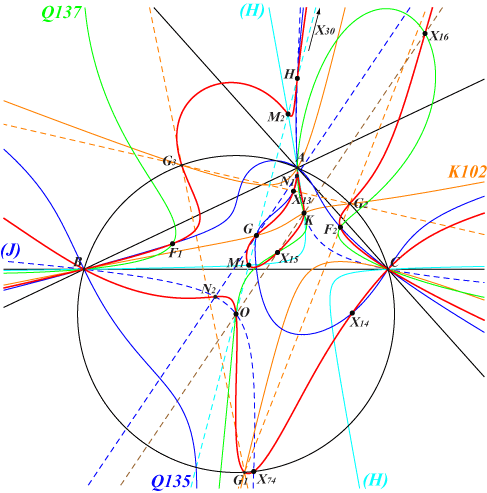too complicated to be written here. Click on the link to download a text file.X(2), X(3), X(4), X(6), X(13), X(14), X(15), X(16), X(30), X(74) points of the Grebe cubic K102 at infinity and on (O) four foci of the Steiner inellipse imaginary foci of the Brocard ellipse other points belowThe quintic Q135 and the quartic Q137 are isogonal transforms of one another. Consider the two decomposed sextics which are the union of Q137 and the Brocard axis, the union of Q135 and the Kiepert hyperbola. These are obviously also isogonal transforms of one another. They generate a pencil of circular sextics which contains two self-isogonal curves. One is the union of K003 and K018, the other is Q138. Q138 has three nodes at A, B, C. It has two isotropic asymptotes passing through X(110), three other asymptotes parallel to those of the Grebe cubic K102 and a 6th asymptote parallel to the Euler line. Q138 meets the circumcircle (O) again at the vertices of the Grebe triangle G1G2G3. See K102 for further properties. Q138 meets the Euler line at X(2), X(3), X(4), X(30) and two other points M1, M2 which lie on the circum-hyperbola (H) with perspector X(3288), passing through X(6), X(95), X(98), X(182), X(183), X(2980), etc. Hence Q138 must contain their isogonal conjugates N1, N2 which lie on the Jerabek hyperbola (J) and on the line passing through X(2), X(511). These four points lie on K763 = pK(X6, X1350). Q138 meets the Thomson cubic K002 at X(2), X(3), X(4), X(6) and seven double points which are A, B, C and the four foci of the Steiner inellipse. Q138 and K002 have the same tangents at these foci. Those at the real foci F1, F2 meet at X(1379), on (O) and on the Brocard axis.